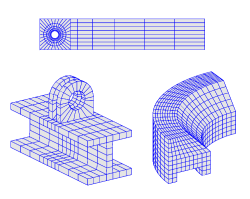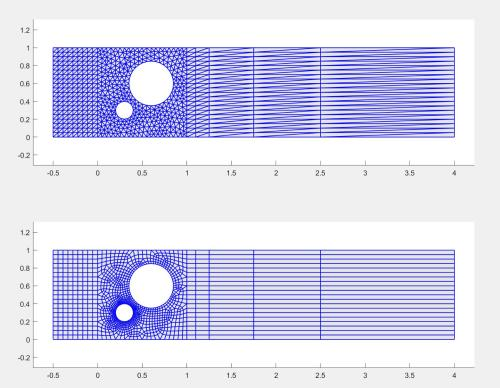gridmerge two automatic unstructured gridsClassicListThreaded6 messagesOpen this post in threaded view
|

gridmerge two automatic unstructured grids

 Hello I'm not quite sure which grid functions to use to be able to stitch two grids together. What I would like to do is a hybrid of two of your grid generation examples. Basically I want to make a grid similar to the flow over a cylinder benchmarkbut I want to make the grid around the circle with gridgen like the rectangle with circular hole from Example 3 I'm interested in doing this because I have multiple staggered cylinders so it would be very difficult for me to use holegrid multiple times and get them to all line up. So my thought was to have a coarse quad mesh for the inlet and outlet and a fine triangle mesh in between all of the cylinders. All of the structured grid examples use gridmerge to merge the two grids, but the grids have to be lined up for it to work. So everything is setup carefully to match. When gridgen is used the grid is unstructured so I don't know where the vertexes on the boundary will be ahead of time. So my question is how do I get the points on the boundary of one grid so I can use gridgen to force those points to be in the next grid that I would like to merge? I have attached a simplified version of what I am hoping to accomplish:GridExample.m As always thank you for your help, Matt
Open this post in threaded view
|

Re: gridmerge two automatic unstructured grids

 Administrator This post was updated on . Dear Matt, Unfortunately, FEATool does not yet support mixing mesh element types (triangles and quadrilaterals, hex and tets etc) but you can use either all quads, or the quad2tri/hex2tet functions to convert a quadrilateral grid to a simplex one. After that it should be fairly straightforward to find the grid point coordinates on edges to join, and use them with rectgrid to create matching grids. And use the findbdr function to find the boundary numbers used by gridmerge . If I have correctly understood what you want to achieve the code example below should give you an idea how one might go about it:% Create center unstructured grid block. r1 = gobj_rectangle( 0, 1, 0, 1, 'R1' ); c1 = gobj_circle( [0.3,0.3], 0.1, 'C1' ); c2 = gobj_circle( [0.6,0.6], 0.25, 'C2' ); geom.objects = { r1, c1, c2 }; geom = geom_apply_formula( geom, 'R1-C1-C2' ); hmax = 0.05; use_quad = true; grid1 = gridgen( geom, 'hmax', hmax, 'gridgen', 'gmsh', 'quad', use_quad ); % Create and merge left/inlet grid. xmin = min(grid1.p(1,:)); ind_p_left_boundary = find( grid1.p(1,:) <= xmin+sqrt(eps) ); y_coord_left_boundary = sort( grid1.p(2,ind_p_left_boundary) ); x_coord_left_boundary = linspace(xmin-0.5,xmin,10); grid2 = rectgrid( x_coord_left_boundary, y_coord_left_boundary ); if( ~use_quad ) grid2 = quad2tri( grid2, 1 ); end ind_bdr1 = findbdr( grid1, ['x<=',num2str(xmin),'+sqrt(eps)'] ); ind_bdr2 = findbdr( grid2, ['x>=',num2str(xmin),'-sqrt(eps)'] ); gridm12 = gridmerge( grid1, ind_bdr1, grid2, ind_bdr2 ); % Create and merge right/outlet grid. xmax = max(grid1.p(2,:)); ind_p_right_boundary = find( grid1.p(1,:) >= xmax-sqrt(eps) ); y_coord_right_boundary = sort( grid1.p(2,ind_p_right_boundary) ); x_coord_right_boundary = xmax + [0,0.1,0.25,0.75,1.5,3]; grid3 = rectgrid( x_coord_right_boundary, y_coord_right_boundary ); if( ~use_quad ) grid3 = quad2tri( grid3, 1 ); end ind_bdrm12 = findbdr( gridm12, ['x>=',num2str(xmax),'-sqrt(eps)'] ); ind_bdr3 = findbdr( grid3, ['x<=',num2str(xmax),'+sqrt(eps)'] ); grid = gridmerge( grid3, ind_bdr3, gridm12, ind_bdrm12 ); % Postprocessing. subplot(2,1,1) plotgrid( grid ) subplot(2,1,2) plotbdr( grid ) is_ok = gridcheck( grid ) == 0
Open this post in threaded view
|

Re: gridmerge two automatic unstructured grids

 Thank you for the help. I'm going to try the triangle mesh using gridgen2d first instead of the quad mesh. I'll see how it goes. Oh if anyone else finds this useful there was a typo in the proposed solution.   % Create and merge right/outlet grid.   xmax = max(grid1.p(2,:)); should be   xmax = max(grid1.p(1,:)); It worked because the example middle grid was a square. Matt
Open this post in threaded view
|

Re: gridmerge two automatic unstructured grids

 Ok I got the mesh setup, but when I try to solve the OpenFOAM button on the GUI is grayed out. If I use the GUI to mesh it again then I can use OpenFOAM solver from the GUI again. I have a feeling there is some sort of problem related to the fact that the grid now has 3 subdomains, but the geometry doesn't. There should only be one subdomain. I tried adding two rectangles, so there were three geometry objects, and I also tried adding the two rectangles to the existing geometry, but even though the geometry shows one object and the boundary mode doesn't show internal boundaries for where the rectangles meet the grid statistics still show 3 subdomains. So my question is how can I find out why the OpenFOAM button is grayed out, and how do I change the grid so there is only 1 subdomain? Thank you for the help, Matt